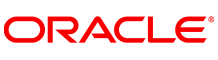New update is available. Click here to update.
Topics

# Minimum Cost to Connect All Points

Moderate0/80
Average time to solve is 30m+2 more companies

## Problem statement

You are given an array, ‘COORDINATES’ that represents the integer coordinates of some points on a 2D plane. Your task is to find the minimum cost to make all the points connected where the cost of connecting two points: (x1, y1) and (x2, y2) is equal to the manhattan distance between them, i.e., |x1 - x2| + |y1 - y2|.

Note:

``````1) An element of the ‘COORDINATES’ array is a pair of ‘X' and ‘Y’ coordinates of a point, i.e., COORDINATES[i] =  (Xi, Yi).

2) |DISTANCE| represents the absolute value of distance.

3) All points are considered to be connected if there is exactly one simple path between two points.

4) According to Wikipedia, a simple path is a path in a plane that does not have repeating points.
``````
Detailed explanation ( Input/output format, Notes, Images )

#### Sample Input 1:

``````2
5
1 4
2 6
8 2
7 12
5 3
4
1 -4
8 6
-5 -3
0 18
``````

#### Sample Output 1:

``````23
44
``````

#### Explanation of Sample Output 1:

``````Test Case 1 :
Connecting (1, 4) and (2, 6) will cost 3.
Connecting (2, 6) and (5, 3) will cost 5.
Connecting (5, 3) and (8, 2) will cost 4.
Connecting (8, 2) and (7, 3) will cost 11.
Total cost = 3 + 5 + 4 + 11 = 23, which is minimum.

Test Case 2 :
Connecting (1, -4) and (-5, -3) will cost 7.
Connecting (-5, -3) and (8, 6) will cost 17.
Connecting (8, 6) and (0, 18) will cost 20.
Total cost = 7 + 17 + 20 = 44, which is minimum.
``````

#### Sample Input 2:

``````2
1
8 -5
3
1004 -754
892 -69664
84510 0
``````

#### Sample Output 2:

``````0
153282
``````Console